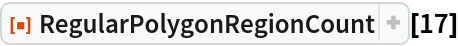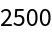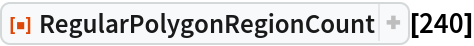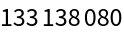Function Repository Resource:

# RegularPolygonRegionCount

Find the number of regions partitioned by the diagonals of a regular polygon

Contributed by: Wolfram|Alpha Math Team
 ResourceFunction["RegularPolygonRegionCount"][n] returns the number of regions formed by the diagonals of a regular polygon with n sides.

## Examples

### Basic Examples (2)

Find the number of regions formed by the diagonals of a regular polygon with 17 sides:

 In:=Out=Find the number of regions formed by the diagonals of a regular polygon with 240 sides:

 In:=Out=## Publisher

Wolfram|Alpha Math Team

## Version History

• 2.0.0 – 23 March 2023
• 1.0.0 – 06 April 2020